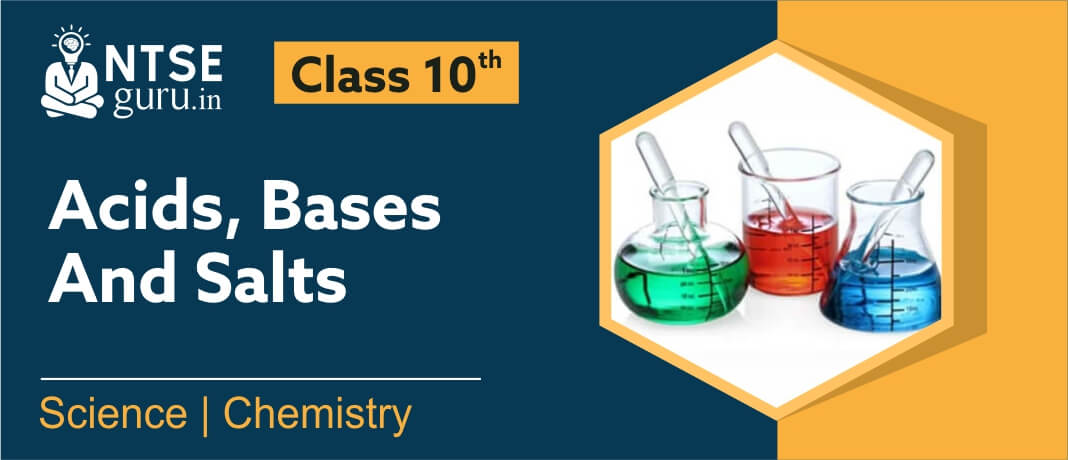# Acid Bases And Salts Class 10Class 10 Science Chapter 2 in NCERT Science deals with Acid, Bases and Salts. The chapter, Acid Bases and Salts class 10 includes all the important concepts about the classification of substances in the mentioned categories and discusses their physical and chemical properties as well. Also, some important salts like common salt, washing soda, baking soda, bleaching powder and plaster of Paris are included and detailed insight into their preparation methods, properties and uses are included in the chapter.

NTSEguru helps you to boost your preparation with the most probable questions in accordance with the revised pattern of X board examination by our expert team of faculties.

Have a great time learning with us!

## Video Lecture - Acid, Bases and Salts Class 10

### Class 10 Science Chapter 2 - Important Questions

1.  When a stain of curry is scrubbed by soap then what change do we observe in the colour of stain and what can we conclude by this observation?

Ans.  Curry contains turmeric which turns red in basic solution. So if on applying soap the colour of stain changes to red, it means soaps are basic in nature.

2.  What is common between hydrangea, Petunia, geranium, turmeric and red cabbage leaves?

Ans.  The extracts of all of these acts as acid base indicators, showing different colours with acids and base.

3.  Complete the following chemical equation & write the names of products:

$$NaOH+Zn\,\,\xrightarrow[{}]{}\_\_\_\_\_\_\_\_\_\_\,+\,{{H}_{2}}$$

Ans.         \begin{align} Â & 2NaOH+Zn\,\,\xrightarrow[{}]{}\,\,\,\,\,\,\,N{{a}_{2}}Zn{{O}_{2}}\,\,\,\,\,\,\,\,\,+{{H}_{2}}\uparrow Â \\Â Â & \,\,\,\,\,\,\,\,\,\,Sodium\,Zincate\,\,\,Hydrogen \\Â \end{align}

4.  What happens when Sodium Carbonate $$(Na_2CO_3)$$) and Sodium Bicarbonate $$(NaHCO_3)$$) react with HCl? Give the chemical reaction involved.

Ans.  When $$(Na_2CO_3)$$) and $$(NaHCO_3)$$) react with HCl, Sodium chloride, carbon dioxide and water are formed.
$$N{{a}_{2}}C{{O}_{3}}+2HCl\to 2NaCl+C{{O}_{2}}+{{H}_{2}}O$$
$$NaHC{{O}_{3}}+HCl\to NaCl+C{{O}_{2}}+{{H}_{2}}O$$

5.  What happens when$$Ca{{\left( OH \right)}_{2}}$$(lime water) reacts with,

i.  $$C{{O}_{2}}$$    ii. Excess of CO2.

Give a complete chemical reaction.

Ans.    (i)  Lime water turns milky due to formation of insoluble calcium carbonate.

$$Ca{{\left( OH \right)}_{2}}+C{{O}_{2}}\xrightarrow{{}}\underset{\begin{smallmatrix} Â Calcium\,carbonate \\ Â \,\,\,\,\,\,\,\,\,(\text{milky}) \end{smallmatrix}}{\mathop{CaC{{O}_{3}}}}\,+{{H}_{2}}O$$

(ii)  On passing excess of CO2, lime water again turns colourless (transparent) due to formation of soluble calcium bicarbonate.

$$CaC{{O}_{3}}+{{H}_{2}}O+C{{O}_{2}}\xrightarrow{{}}\underset{\begin{smallmatrix} \text{Calcium}\,\text{bi}\,\text{carbonate} \\ \,\,\,\,\,\,\,\,\,\,\,\,\,\,\,\text{(soluble)} \end{smallmatrix}}{\mathop{Ca{{\left( HC{{O}_{3}} \right)}_{2}}}}\,$$

6.  Phenolphthalein is added to dil. NaOH solution in a test tube. Colour of the solution changes to pink. Now if dil. HCl is added drop by drop in the test tube. What will you observe and why?

Ans. If dilute HCl is added drop by drop, then at one point, the pink colour in the solution disappears. At this point, the sodium hydroxide solution in the test tube has completely reacted (neutralized) with the hydrochloric acid added. The following neutralization reaction has taken place.

$$NaOH+HCl\xrightarrow{{}}\underset{Sodium\,\,Chloride}{\mathop{NaCl}}\,+{{H}_{2}}O$$

7.  When copper oxide is treated with dil. HCl then the colour of solution changes. Why?

Ans.   Copper oxide on reacting with dil. HCl forms copper chloride and H2O. Copper chloride is bluish green in colour so, the colour of solution changes.

\begin{align} Â & CuO+2HCl\to CuC{{l}_{2}}\left( aq \right)+{{H}_{2}}O \\ Â & Bluish\,green \\ \end{align}

8.  Compare the properties of acids and bases.

Ans.

 Acid Bases Acids are sour in taste, generally corrosive in nature. Bases are bitter in taste. Strong bases are corrosive in nature. They turn blue litmus to red, phenolphthalein remains colourless in acidic solution. Bases turn red litmus blue & give pink colour with phenolphthalein indicator. On reacting with metals, acids liberate H2 gas. Generally, bases do not react with metals. Strong Bases react with some metals like Zn & Al to liberate H2 gas. On reacting with metal carbonates & bicarbonates, acids liberate CO2 gas. Bases do not react with metal carbonates & bicarbonates. Solutions of acids conduct electricity. Solutions of bases also conduct electricity. Acids release H+ ions in aqueous solutions. Bases release $$O{{H}^{-}}$$ions in aqueous solutions.

## Related Posts

X Board exams play a very important life in building the foundation of a student’s dynamic career and it is very imp...

Gender, Religion and Caste is an important chapter of Civics Class 10. It is Chapter 4 of your ...